# How to Enlarge a Shape

How to Enlarge a Shape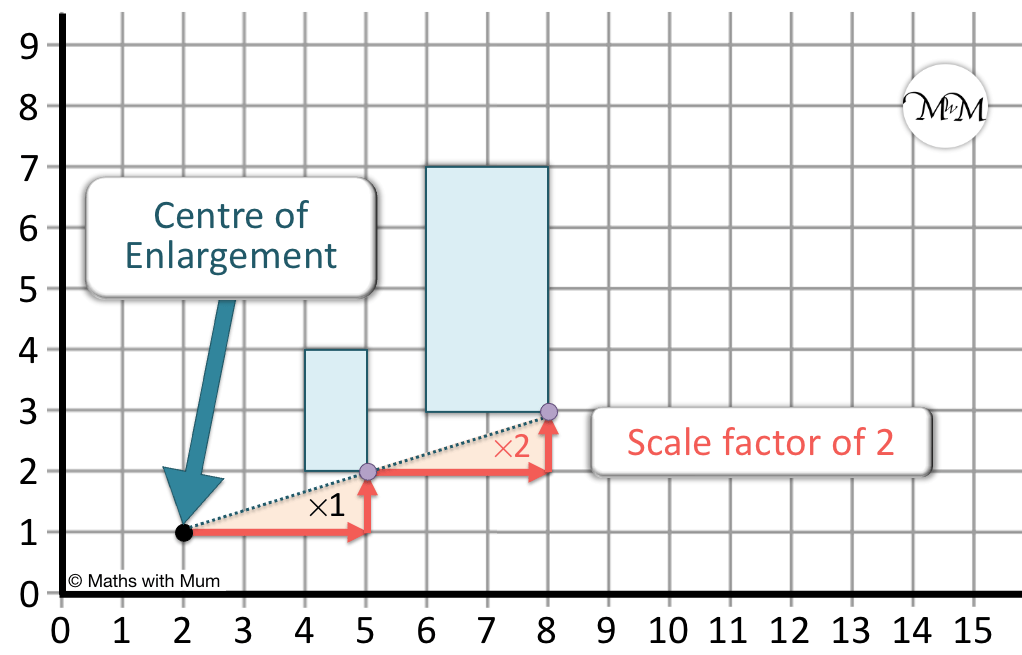• Enlarging a shape means to multiply the lengths of each side by the same amount to create a new image.
• The scale factor tells us how many times larger the new shape will be and how many times further away it will be from the centre of enlargement.
• The centre of enlargement in this example is (2, 1) and the scale factor is 2.
• This means that the sides of the image are all 2 times as large as the original object.
• To enlarge a shape, we move all of its corners away from the centre of enlargement by multiplying the distance by the scale factor.
• The original object has a corner that is 3 to the right and 1 up from the centre of enlargement.
• The scale factor is two, so the new corner will be twice as far away.
• The corresponding corner of the image is now 6 to the right and 2 up from the centre of enlargement.
Enlarging a shape means to change its size.

Count how far away the corners of the shape are from the centre of enlargement and multiply these distances by the scale factor.• The scale factor is 2, so all corners will be moved twice as far away from the centre of enlargement.
• The first corner is across 2 and up 1, so multiplying this by 2, the new corner is across 4 and up 2.
• The second corner is across 3 and up 1, so multiplying this by 2, the new corner is across 6 and up 2.
• The third corner is across 2 and up 3, so multiplying this by 2, the new corner is across 4 and up 6.
• The fourth corner is across 3 and up 3, so multiplying this by 2, the new corner is across 6 and up 6.
• The lengths of the new image are all twice as long as the original object.
• We can check our answer by drawing straight lines from the centre of enlargement through the corners.
• All of the lines should pass through the centre of enlargement and both the corners of the object and the enlarged image.Supporting Lessons

#### Enlarging Shapes Video Accompanying Activity Sheets# Enlarging Shapes

## How to Enlarge a Shape

Enlarging a shape means to change its size. To enlarge a shape, multiply all lengths of the shape by the scale factor. The scale factor tells us how many times larger the shape will be.

For example, enlarging a shape by scale factor 2 means that all of the sides will become 2 times longer.This rectangle is 1 square wide and 2 squares long.

Multiplying these lengths by the scale factor of 2, the new image will be 2 squares wide and 4 squares long.

Here is an example of multiplying a triangle by scale factor 2.

To enlarge a triangle with sloping sides, it is easiest to enlarge the base and the height.The base is 2 squares long and the height is 3 squares long.

Multiply these lengths by the scale factor of 2 to find the enlarged shape.

The base is now 4 squares long and the height is 6 squares long.

The triangle can be drawn by connecting the top of the height to each end of the base.

Here is an example of enlarging a shape by scale factor 3. This means that all of the sides will be three times longer.The original rectangle has width 1 and length 2.

Multiplying these lengths by 3, the new rectangle image has width 3 and length 6.

Here is another example of enlarging a shape by scale factor 3.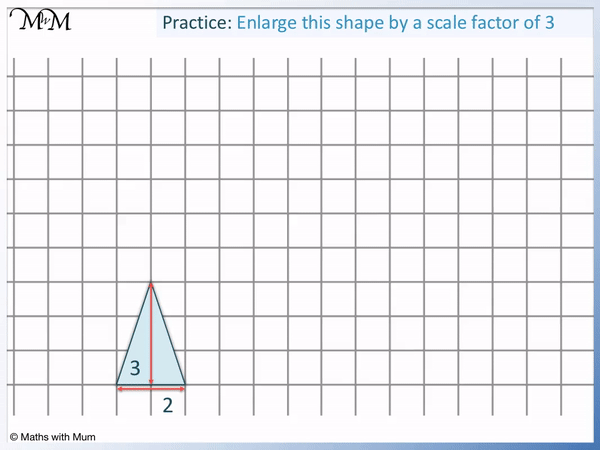The original triangle object has a base of 2 and a height of 3.

Multiplying these lengths by 3, the new triangle image has a base of 6 and a height of 9.

## How to Enlarge a Shape by a Scale Factor

To enlarge a shape by a scale factor, first count the distances of each point on the shape from the centre of enlargement. Multiply each distance by the scale factor to find its new position. For example, if a point is 2 to the right and 1 up from the centre of enlargement and the scale factor is 2, the new position will be 4 to the right and 2 up from the centre of enlargement.

For example, enlarge the following triangle by scale factor 3 about the centre of enlargement.

The topmost corner is 3 right and 1 down from the centre of enlargement. Multiply these distances by the scale factor to find the new position of this corner.

3 × 3 = 9 and so, the new corner will be 9 to the right of the centre of enlargement.

1 × 3 = 3 and so, the new corner will be 3 down from the centre of enlargement.

The corner moved from 3 right, 1 down to be 9 right, 3 down.By multiplying all distances by 3, we see the following distances to the enlarged shape.

Original Object Corner Scale Factor of 3 New Image Corner
3 right, 1 down × 3 9 right, 3 down
2 right, 3 down × 3 6 right, 9 down
4 right, 3 down × 3 12 right, 9 down

Here is another example of enlarging a shape about a point.

This time the scale factor is 2 and so, all distances have been multiplied by 2.The following table shows the positions of the new corners of the enlarged shape.

Original Object Corner Scale Factor of 2 New Image Corner
1 right, 1 down × 2 2 right, 2 down
2 right, 1 down × 2 4 right, 2 down
2 right, 4 down × 2 4 right, 8 down
0 right, 4 down × 2 0 right, 8 down
0 right, 3 down × 2 0 right, 6 down
1 right, 3 down × 2 2 right, 6 down

Once all of the corners of the enlarged shape have been put in position, the shape can be drawn by connecting the corners.

## How to Find the Centre of Enlargement

The centre of enlargement is the coordinate about which a shape is enlarged. To find the centre of enlargement, draw straight lines from each corner of the enlarged shape back through the corners of the original shape. The centre of enlargement is the point where all of the lines cross over.

We will find the centre of enlargement of the example below.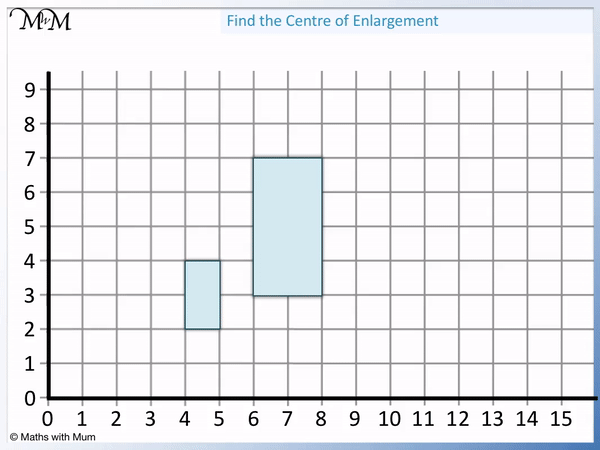The first step is to find two corresponding corners. We have marked each of the corresponding corners in the same colour.

Use a ruler to draw a straight line between the corner of the enlarged shape and the same corner on the original object.

Continue this straight line far past the original object.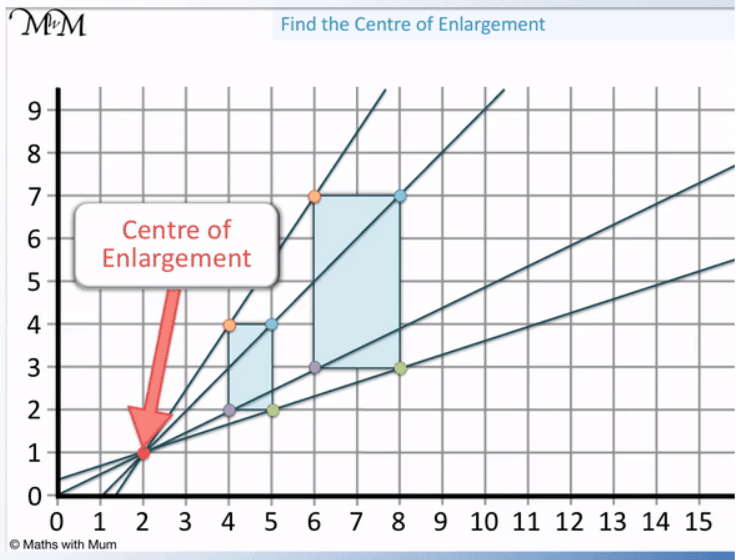Once you have repeated this for two corners, the two straight lines drawn should cross over. This is the position of the centre of enlargement.

We can check our answer by drawing lines through more of the corners and checking that they all cross over in the same position.

In this example, the centre of enlargement is at (2, 1).

## How to Find the Scale Factor of Enlargement

To find the scale factor of enlargement, find two corresponding sides that match up on the original shape and the enlarged shape. Divide the longer side by the shorter side to find the scale factor of enlargement.

For example, in the shape below, two corresponding sides are marked. These are both the rightmost sides of the shape.

One side is 2 squares long and the other side is 6 squares long.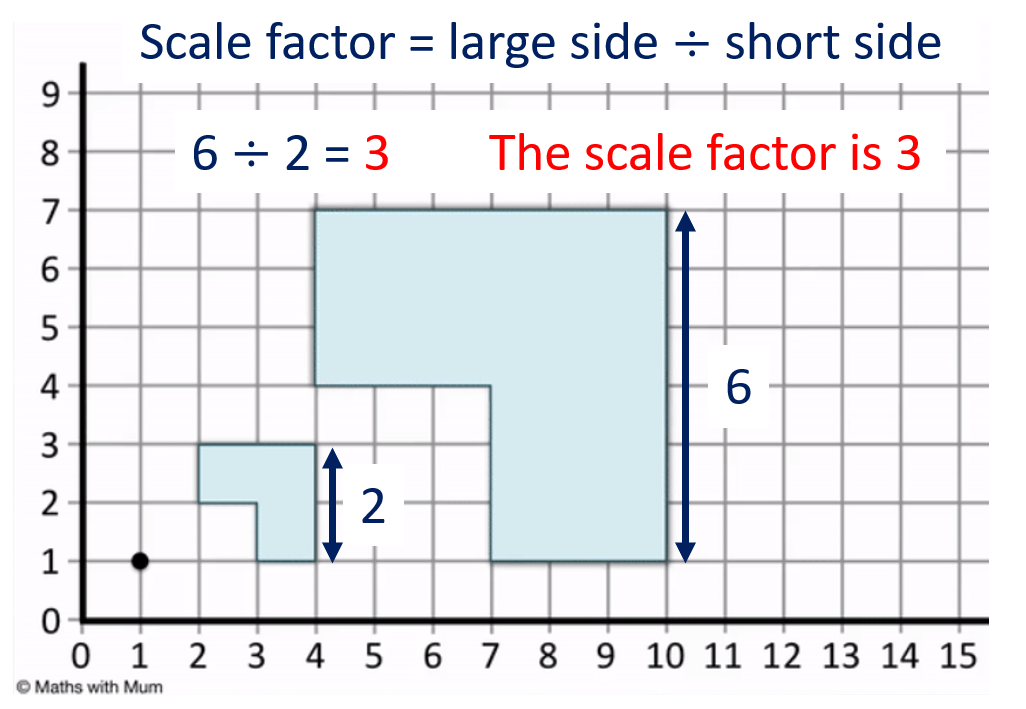To find the scale factor of enlargement, divide the longer side by the short side.

6 ÷ 2 = 3 and so, the scale factor is 3.

This means that all of the sides on the smaller shape have been multiplied by 3.

It also means that all of the sides on the larger shape can be divided by 3 to make the smaller shape.

We can check this answer by repeating this process for any of the other corresponding sides.

For example, the bottom side of the larger shape is 3 long and the bottom side of the smaller shape is 1 long.

3 ÷ 1 = 3 and this confirms our answer of the scale factor being equal to 3.Now try our lesson on Drawing and Interpreting Pictograms where we learn how to draw pictorgrams.